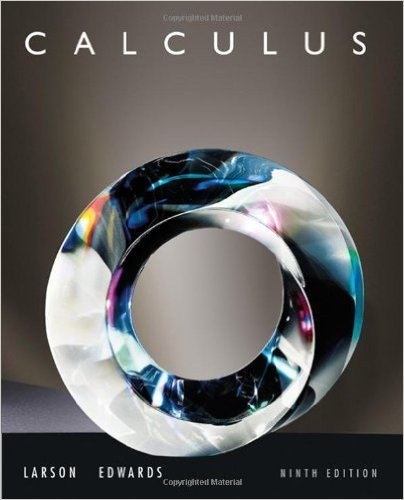×
×

# Solutions for Chapter P4: Fitting Models to Data## Full solutions for Calculus | 9th Edition

ISBN: 9780547167022Solutions for Chapter P4: Fitting Models to Data

Solutions for Chapter P4
4 5 0 421 Reviews
15
4
##### ISBN: 9780547167022

Calculus was written by and is associated to the ISBN: 9780547167022. This expansive textbook survival guide covers the following chapters and their solutions. This textbook survival guide was created for the textbook: Calculus , edition: 9. Chapter P4: Fitting Models to Data includes 21 full step-by-step solutions. Since 21 problems in chapter P4: Fitting Models to Data have been answered, more than 68439 students have viewed full step-by-step solutions from this chapter.

Key Calculus Terms and definitions covered in this textbook

P(A or B) = P(A) + P(B) - P(A and B). If A and B are mutually exclusive events, then P(A or B) = P(A) + P(B)

• Combination

An arrangement of elements of a set, in which order is not important

• Conic section (or conic)

A curve obtained by intersecting a double-napped right circular cone with a plane

• Equivalent equations (inequalities)

Equations (inequalities) that have the same solutions.

• Expanded form

The right side of u(v + w) = uv + uw.

• Half-life

The amount of time required for half of a radioactive substance to decay.

• Horizontal translation

A shift of a graph to the left or right.

• Hyperbola

A set of points in a plane, the absolute value of the difference of whose distances from two fixed points (the foci) is a constant.

• Limaçon

A graph of a polar equation r = a b sin u or r = a b cos u with a > 0 b > 0

• Logarithm

An expression of the form logb x (see Logarithmic function)

• Measure of center

A measure of the typical, middle, or average value for a data set

• Monomial function

A polynomial with exactly one term.

• Natural logarithmic regression

A procedure for fitting a logarithmic curve to a set of data.

• Obtuse triangle

A triangle in which one angle is greater than 90°.

• Parameter

See Parametric equations.

• Reference angle

See Reference triangle

• Second

Angle measure equal to 1/60 of a minute.

• Semiperimeter of a triangle

One-half of the sum of the lengths of the sides of a triangle.

• Variance

The square of the standard deviation.

• Vertex of a parabola

The point of intersection of a parabola and its line of symmetry.

×